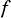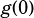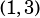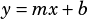How do you do Test 9 section 3 number 20?

This question is about perpendicular lines, so the first thing to remember is that perpendicular lines have negative reciprocal slopes. The graph of, shown, has a slope of -2. That means the slope of, not shown, must have a slope of.

The second thing to quickly note is that this question is using function notation and asking for. That’s the same as asking for the-intercept; it’s just the question 20 way to ask it.

We know thatpasses through, and we know it has a slope of, so all we really need to do is count leftwards.is only 1 unit away from the-axis, and when you have a slope of, you travel 0.5 units down when you travel 1 unit left. Therefore, the-intercept—aka—is 2.5.

If you like algebra better, you can plug the slope and the point you know into slope-intercept form () and solve for: